# Can I get a solution for this question? It's quite hard to solve. There is no reference about and I don't get w...

Can I get a solution for this question? It's quite hard to solve.

There is no reference aboutand I don't get what this question's purpose is.

Is alpha the alpha from bernoulli's equation?

Question:

An approximate equation for the velocity distribution in a pipe with turbulent flow is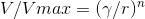where Vmax is the centerline velocity,is the distance from the wall of the pipe, r is the radius of the pipe, and n is an exponent that depends on the Reynolds number and varies between 1/6 and 1/8 for most applications. Derive a formula foras a function of n. What isif n=1/7?

V/Vmax (/r" G/r)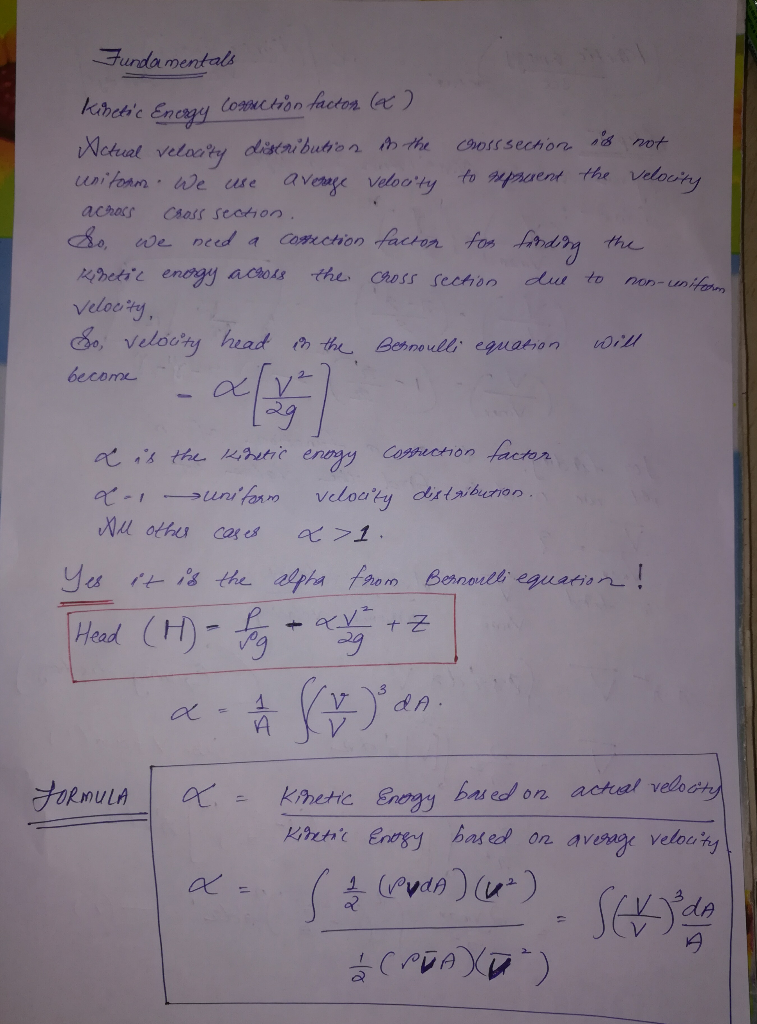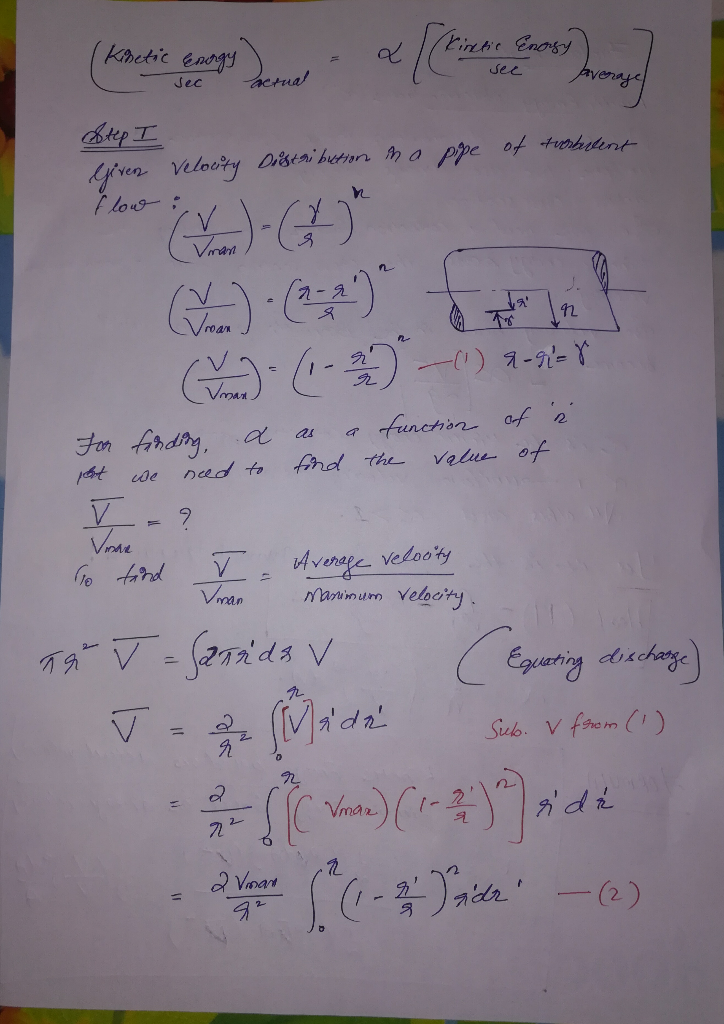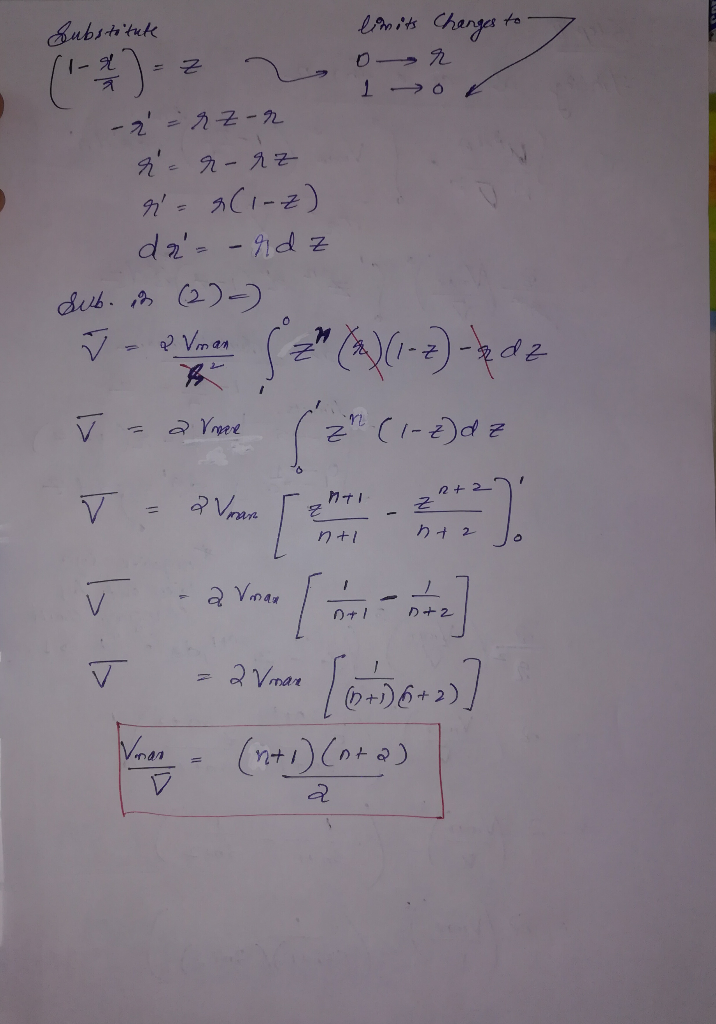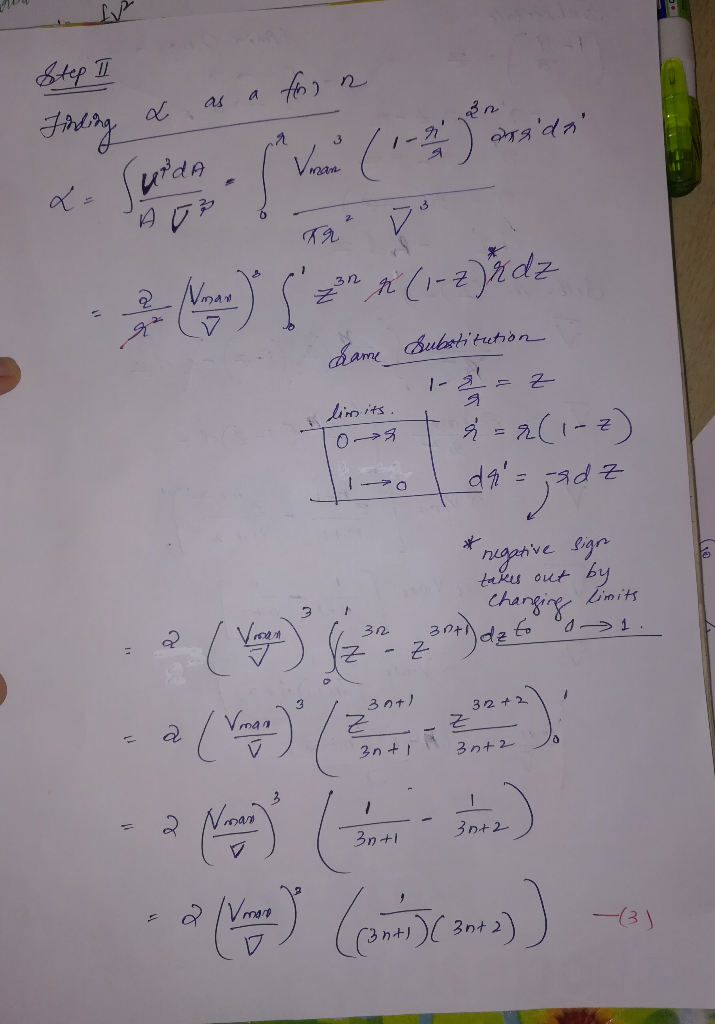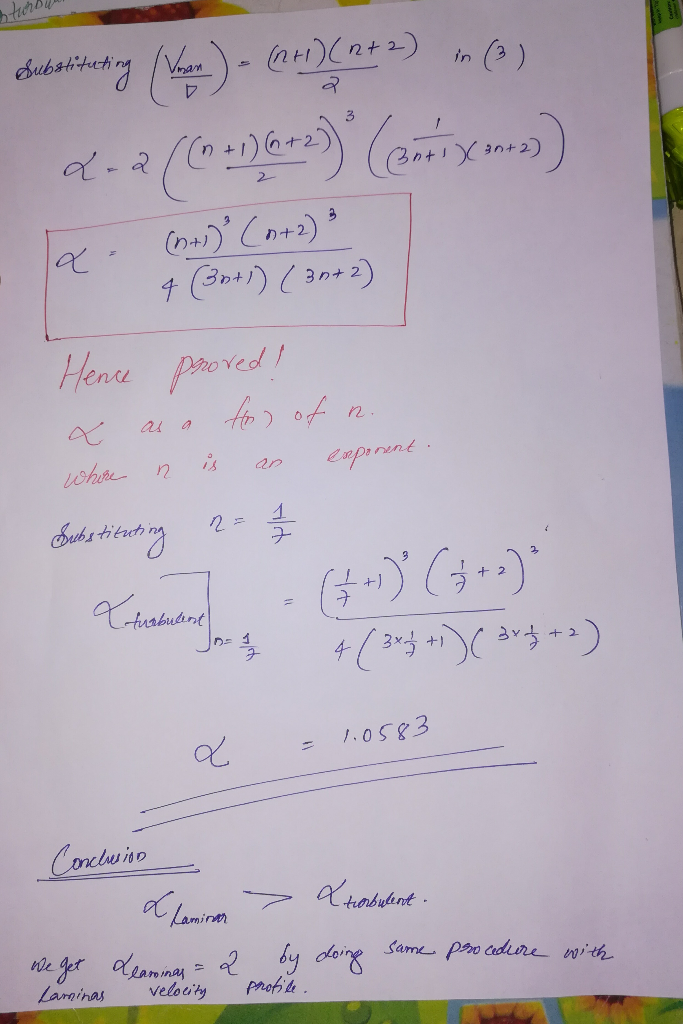##### Add Answer of: Can I get a solution for this question? It's quite hard to solve. There is no reference about and I don't get w...
More Homework Help Questions Additional questions in this topic.